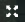# Introduction

To view the lesson in full screen mode, click on thebutton.

Being “good at math” often referred to knowing basic facts quickly. But is that what defines a mathematically proficient student? What about the other skills—measurement, angles, fractions, time, etc.”? What role does knowing the basic addition and subtraction facts playing in learning other content?

This course will examine the trajectory, or journey, of students learning the basic addition and subtraction facts.

• Preview of Course Outline
• When are students expected to master basic multiplication facts?
• Multiplication and division situations
• What are basic facts and why master them?
• Fluency and memorization
• 3 Phases to Mastery
• Sequencing specific multiplication strategies
• Connecting to subtraction
• Progress Monitoring and Assessing Mastery
• Lasting Thoughts